Next: Hyperplane Transformations Up: Hyperplanes and Duality Previous: The Duality Principle

### Desargues Theorem

Projective geometry was invented by the French mathematician Desargues (1591-1661) (for a biography in French, see http://bib1.ulb.ac.be/coursmath/bio/desargue.htm). One of his theorems is considered to be a cornerstone of the formalism. It states that Two triangles are in perspective from a point if and only if they are in perspective from a line'' (see fig. 2.1):

Theorem: Let A, B, C and A', B, C' be two triangles in the (projective) plane. The lines AA', BB', CC' intersect in a single point if and only if the intersections of corresponding sides (AB, A'B'), (BC, B'C'), (CA, C'A') lie on a single line.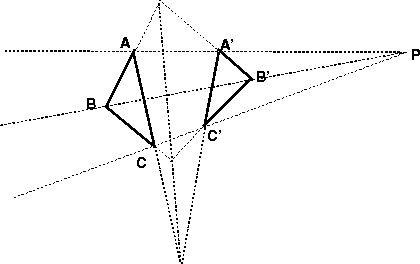The theorem has a clear self duality: given two triplets of lines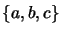and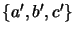defining two triangles, the intersections of the corresponding sides lie on a line if and only if the lines of intersection of the corresponding vertices intersect in a point.

We will give an algebraic proof: Let P be the common intersection of AA', BB', CC'. Hence there are scalars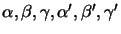such that: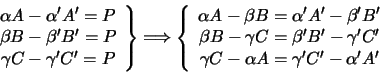This indicates that the point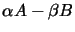on the line AB also lies at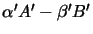on the line A'B', and hence corresponds to the intersection of AB and A'B', and similarly for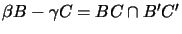and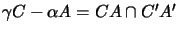. But given that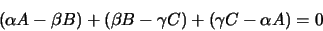the three intersection points are linearly dependent, i.e. collinear.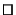Exercise 2.4   :  The sun (viewed as a point light source) casts on the planar ground the shadow A'B'C' of a triangular roof ABC (see fig. 2.2). Consider a perspective image of all this, and show that it is a Desargueian configuration. To which 3D line does the line of intersections in Desargues theorem correspond? If a further point D in the plane ABC produces a shadow D', show that it is possible to reconstruct the image of D from that of D'.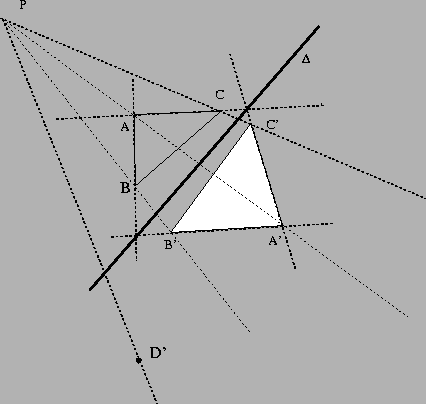Next: Hyperplane Transformations Up: Hyperplanes and Duality Previous: The Duality Principle
Bill Triggs
1998-11-13